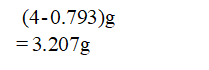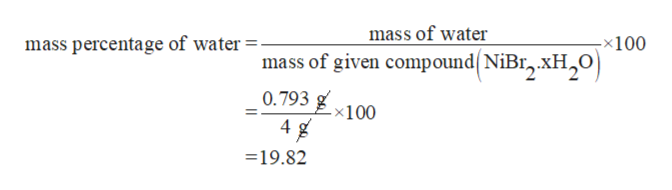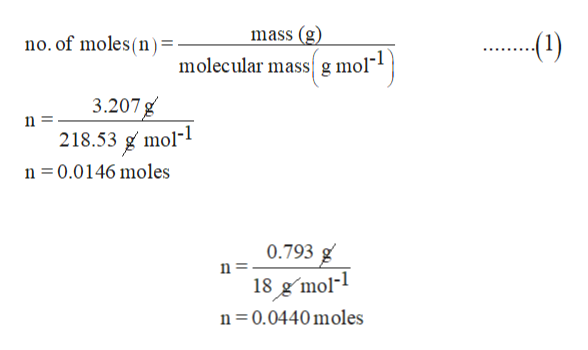# A 4.00 gram sample of hydrate of nickel(II) bromide loses 0.793 grams of water when heated. Determine the mass percent water in the hydrate and the formula of the hydrate

Question
57 views

A 4.00 gram sample of hydrate of nickel(II) bromide loses 0.793 grams of water when heated. Determine the mass percent water in the hydrate and the formula of the hydrate

check_circle

Step 1

Mass of hydrated nickel bromide = 4 g

When it heated it lose water of 0.793 g from nickel bromide.

Mass of nickel bromide without water moleculesStep 2

The mass percentage is calculated as the mass of a component divided by the total mass of the mixture and then multiply by 100.

The mass percentage of water is 19.82.help_outlineImage Transcriptionclosemass of water mass percentage of water -x100 mass of given compound(NiBr,.XH2O 0.793 4 -x100 19.82 fullscreen
Step 3

Mass of anhydrous nickel bromide is 3.207g.

So no. of the mole of anhydrous nickel bromide

By using equation (1), no...help_outlineImage Transcriptionclosemass (g (1) no. of moles(n) = molecular massg mol 3.207g 218.53 g mol- n 0.0146 moles n = 0.793 g n = 18 gmol- n 0.0440moles fullscreen

### Want to see the full answer?

See Solution

#### Want to see this answer and more?

Solutions are written by subject experts who are available 24/7. Questions are typically answered within 1 hour.*

See Solution
*Response times may vary by subject and question.
Tagged in

### Other